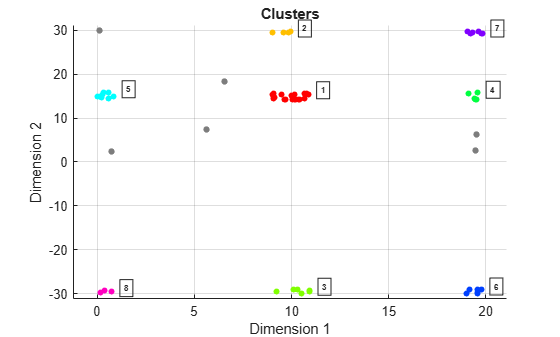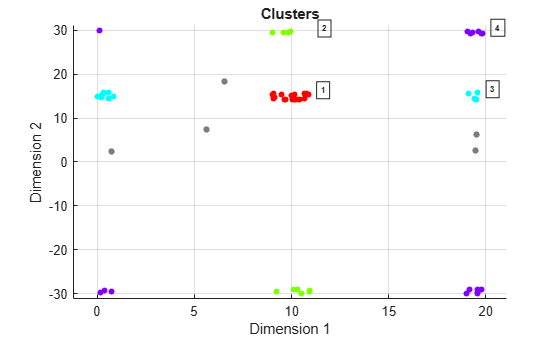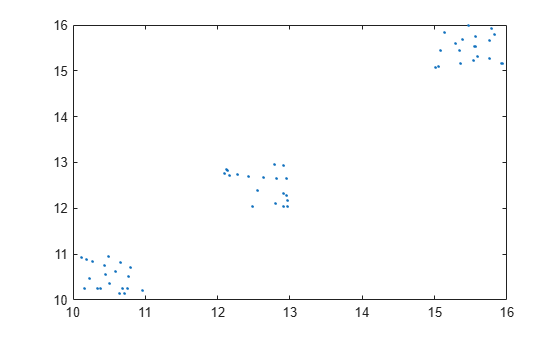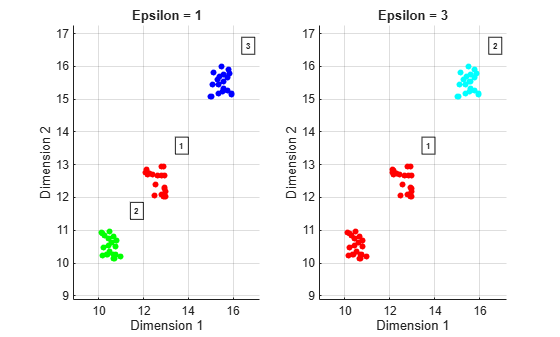# clusterDBSCAN

Density-based algorithm for clustering data

## Description

`clusterDBSCAN` clusters data points belonging to a P-dimensional feature space using the density-based spatial clustering of applications with noise (DBSCAN) algorithm. The clustering algorithm assigns points that are close to each other in feature space to a single cluster. For example, a radar system can return multiple detections of an extended target that are closely spaced in range, angle, and Doppler. `clusterDBSCAN` assigns these detections to a single detection.

• The DBSCAN algorithm assumes that clusters are dense regions in data space separated by regions of lower density and that all dense regions have similar densities.

• To measure density at a point, the algorithm counts the number of data points in a neighborhood of the point. A neighborhood is a P-dimensional ellipse (hyperellipse) in the feature space. The radii of the ellipse are defined by the P-vector ε. ε can be a scalar, in which case, the hyperellipse becomes a hypersphere. Distances between points in feature space are calculated using the Euclidean distance metric. The neighborhood is called an ε-neighborhood. The value of ε is defined by the `Epsilon` property. `Epsilon` can either be a scalar or P-vector:

• A vector is used when different dimensions in feature space have different units.

• A scalar applies the same value to all dimensions.

• Clustering starts by finding all core points. If a point has a sufficient number of points in its ε-neighborhood, the point is called a core point. The minimum number of points required for a point to become a core point is set by the `MinNumPoints` property.

• The remaining points in the ε-neighborhood of a core point can be core points themselves. If not, they are border points. All points in the ε-neighborhood are called directly density reachable from the core point.

• If the ε-neighborhood of a core point contains other core points, the points in the ε-neighborhoods of all the core points merge together to form a union of ε-neighborhoods. This process continues until no more core points can be added.

• All points in the union of ε-neighborhoods are density reachable from the first core point. In fact, all points in the union are density reachable from all core points in the union.

• All points in the union of ε-neighborhoods are also termed density connected even though border points are not necessarily reachable from each other. A cluster is a maximal set of density-connected points and can have an arbitrary shape.

• Points that are not core or border points are noise points. They do not belong to any cluster.

• The `clusterDBSCAN` object can estimate ε using a k-nearest neighbor search, or you can specify values. To let the object estimate ε, set the `EpsilonSource` property to `'Auto'`.

• The `clusterDBSCAN` object can disambiguate data containing ambiguities. Range and Doppler are examples of possibly ambiguous data. Set `EnableDisambiguation` property to `true` to disambiguate data.

To cluster detections:

1. Create the `clusterDBSCAN` object and set its properties.

2. Call the object with arguments, as if it were a function.

## Creation

### Syntax

``clusterer = clusterDBSCAN``
``clusterer = clusterDBSCAN(Name,Value)``

### Description

````clusterer = clusterDBSCAN` creates a `clusterDBSCAN` object, `clusterer`, object with default property values.Effect of Epsilon on Clustering```
````clusterer = clusterDBSCAN(Name,Value)` creates a `clusterDBSCAN` object, `clusterer`, with each specified property `Name` set to the specified `Value`. You can specify additional name-value pair arguments in any order as (`Name1`,`Value1`,...,`NameN`,`ValueN`). Any unspecified properties take default values. For example, clusterer = clusterDBSCAN('MinNumPoints',3,'Epsilon',2, ... 'EnableDisambiguation',true,'AmbiguousDimension',[1 2]); creates a clusterer with the `EnableDisambiguation` property set to true and the `AmbiguousDimension` set to `[1,2]`.```

## Properties

expand all

Unless otherwise indicated, properties are nontunable, which means you cannot change their values after calling the object. Objects lock when you call them, and the `release` function unlocks them.

If a property is tunable, you can change its value at any time.

Source of epsilon values defining an ε-neighborhood, specified as `'Property'` or `'Auto'`.

• When you set the `EpsilonSource` property to `'Property'`, ε is obtained from the `Epsilon` property.

• When you set the `EpsilonSource` property to `'Auto'`, ε is estimated automatically using a k-nearest neighbor (k-NN) search over a range of k values from kmin to kmax.

`$\begin{array}{l}{k}_{\mathrm{min}}=\text{MinNumPoints}-1\\ {k}_{\mathrm{max}}=\text{MaxNumPoints}-1\\ \end{array}$`

The subtraction of one is needed because the number of neighbors of a point does not include the point itself, whereas `MinNumPoints` and `MaxNumPoints` refer to the total number of points in a neighborhood.

Data Types: `char` | `string`

Radius for a neighborhood search, specified as a positive scalar or positive, real-valued 1-by-P row vector. P is the number of features in the input data, `X`.

`Epsilon` defines the radii of an ellipse around any point to create an ε-neighborhood. When `Epsilon` is a scalar, the same radius applies to all feature dimensions. You can apply different epsilon values for different features by specifying a positive, real-valued 1-by-P row vector. A row vector creates a multidimensional ellipse (hyperellipse) search area, useful when the data features have different physical meanings, such as range and Doppler. See Estimate Epsilon for more information about this property.

You can use the `clusterDBSCAN.estimateEpsilon` or `clusterDBSCAN.discoverClusters` object functions to help estimate a scalar value for epsilon.

Example: `[11 21.0]`

Tunable: Yes

#### Dependencies

To enable this property, set the `EpsilonSource` property to `'Property'`.

Data Types: `double`

Minimum number of points in an ε-neighborhood of a point for that point to become a core point, specified as a positive integer. See Choosing the Minimum Number of Points for more information. When the object automatically estimates epsilon using a k-NN search, the starting value of k (kmin) is `MinNumPoints` - 1.

Example: `5`

Data Types: `double`

Set end of k-NN search range, specified as a positive integer. When the object automatically estimates epsilon using a k-NN search, the ending value of k (kmax) is `MaxNumPoints` - 1.

Example: `13`

#### Dependencies

To enable this property, set the `EpsilonSource` property to `'Auto'`.

Data Types: `double`

Length of the stored epsilon history, specified as a positive integer. When set to one, the history is memory-less, meaning that each epsilon estimate is immediately used and no moving-average smoothing occurs. When greater than one, epsilon is averaged over the history length specified.

Example: `5`

#### Dependencies

To enable this property, set the `EpsilonSource` property to `'Auto'`.

Data Types: `double`

Switch to enable disambiguation of dimensions, specified as `false` or `true`. When `true`, clustering can occur across boundaries defined by the input `amblims` at execution. Use the `AmbiguousDimensions` property to specify the column indices of `X` in which ambiguities can occur. You can disambiguate up to two dimensions. Turning on disambiguation is not recommended for large data sets.

Data Types: `logical`

Indices of ambiguous dimensions, specified as a positive integer or 1-by-2 vector of positive integers. This property specifies the column of `X` in which to apply disambiguation. A positive integer indicates a single ambiguous dimension in the input data matrix `X`. A 1-by-2 row vector specifies two ambiguous dimensions. The size and order of `AmbiguousDimension` must be consistent with the object input `amblims`.

Example: `[3 4]`

#### Dependencies

To enable this property, set the `EnableDisambiguation` property to `true`.

Data Types: `double`

## Usage

### Syntax

``idx = clusterer(X)``
``[idx,clusterids] = clusterer(X)``
``[___] = clusterer(X,amblims)``
``[___] = clusterer(X,update)``
``[___] = clusterer(X,amblims,update)``

### Description

example

````idx = clusterer(X)` clusters the points in the input data, `X`. `idx` contains a list of IDs identifying the cluster to which each row of `X` belongs. Noise points are assigned as '–1'.```

example

````[idx,clusterids] = clusterer(X)` also returns an alternate set of cluster IDs, `clusterids`, for use in the `phased.RangeEstimator` and `phased.DopplerEstimator` objects. `clusterids` assigns a unique ID to each noise point.```
````[___] = clusterer(X,amblims)` also specifies the minimum and maximum ambiguity limits, `amblims`, to apply to the data.To enable this syntax, set the `EnableDisambiguation` property to `true`.```
````[___] = clusterer(X,update)` automatically estimates epsilon from the input data matrix, `X`, when `update` is set to `true`. The estimation uses a k-NN search to create a set of search curves. For more information, see Estimate Epsilon. The estimate is an average of the L most recent Epsilon values where L is specified in `EpsilonHistoryLength` To enable this syntax, set the `EpsilonSource` property to `'Auto'`, optionally set the `MaxNumPoints` property, and also optionally set the `EpsilonHistoryLength` property.```
````[___] = clusterer(X,amblims,update)` sets ambiguity limits and estimates epsilon when `update` is set to `true`. To enable this syntax, set `EnableDisambiguation` to `true` and set `EpsilonSource` to `'Auto'`.```

### Input Arguments

expand all

Input feature data, specified as a real-valued N-by-P matrix. The N rows correspond to feature points in a P-dimensional feature space. The P columns contain the values of the features over which clustering takes place. The DBSCAN algorithm can cluster any type of data with appropriate `MinNumPoints` and `Epsilon` settings. For example, a two-column input can contain the xy Cartesian coordinates, or range and Doppler.

Data Types: `double`

Ambiguity limits, specified as a real-valued 1-by-2 vector or real-valued 2-by-2 matrix. For a single ambiguity dimension, specify the limits as a 1-by-2 vector [MinAmbiguityLimitDimension1,MaxAmbiguityLimitDimension1]. For two ambiguity dimensions, specify the limits as a 2-by-2 matrix [MinAmbiguityLimitDimension1, MaxAmbiguityLimitDimension1; MinAmbiguityLimitDimension2,MaxAmbiguityLimitDimension2]. Ambiguity limits allow clustering across boundaries to ensure that ambiguous detections are appropriately clustered.

The ambiguous columns of `X` are defined in the `AmbiguousDimension` property. `amblims` defines the minimum and maximum ambiguity limits in the same units as the data in the `AmbiguousDimension` columns of `X`.

Example: `[0 20; -40 40]`

#### Dependencies

To enable this argument, set `EnableDisambiguation` to `true` and set the `AmbiguousDimension` property.

Data Types: `double`

Enable automatic update of the epsilon estimate, specified as `false` or `true`.

• When `true`, the epsilon threshold is first estimated as the average of the knees of k-NN search curves. The estimate is then added to a buffer whose length L is set in the `EpsilonHistoryLength` property. The final epsilon that is used is calculated as the average of the L-length epsilon history buffer. If `EpsilonHistoryLength` is set to `1`, the estimate is memory-less. Memory-less means that each epsilon estimate is immediately used and no moving-average smoothing occurs.

• When `false`, a previous epsilon estimate is used. Estimating epsilon is computationally intensive and not recommended for large data sets.

#### Dependencies

To enable this argument, set the `EpsilonSource` property to `'Auto'` and specify the `MaxNumPoints` property.

Data Types: `double`

### Output Arguments

expand all

Cluster indices, returned as an integer-valued N-by-1 column vector. `idx` represents the clustering results of the DBSCAN algorithm. Positive `idx` values correspond to clusters that satisfy the DBSCAN clustering criteria. A value of '`-1`' indicates a DBSCAN noise point.

Data Types: `double`

Alternative cluster IDs, returned as a 1-by-N row vector of positive integers. Each value is a unique identifier indicating a hypothetical target cluster. This argument contains unique positive cluster IDs for all points including noise. In contrast, the `idx` output argument labels noise points with '–1'. Use `clusterids` as the input to Phased Array System Toolbox™ objects such as `phased.RangeEstimator` and `phased.DopplerEstimator`.

Data Types: `double`

## Object Functions

To use an object function, specify the System object™ as the first input argument. For example, to release system resources of a System object named `obj`, use this syntax:

`release(obj)`

expand all

 `clusterDBSCAN.discoverClusters` Find cluster hierarchy in data `clusterDBSCAN.estimateEpsilon` Estimate neighborhood clustering threshold `clusterDBSCAN.plot` Plot clusters
 `step` Run System object algorithm `release` Release resources and allow changes to System object property values and input characteristics `reset` Reset internal states of System object

## Examples

collapse all

Create detections of extended objects with measurements in range and Doppler. Assume the maximum unambiguous range is 20 m and the unambiguous Doppler span extends from $-30$ Hz to $30$ Hz. Data for this example is contained in the `dataClusterDBSCAN.mat` file. The first column of the data matrix represents range, and the second column represents Doppler.

The input data contains the following extended targets and false alarms:

• an unambiguous target located at $\left(10,15\right)$

• an ambiguous target in Doppler located at$\left(10,-30\right)$

• an ambiguous target in range located at $\left(20,15\right)$

• an ambiguous target in range and Doppler located at $\left(20,30\right)$

• 5 false alarms

Create a `clusterDBSCAN` object and specify that disambiguation is not performed by setting `EnableDisambiguation` to `false`. Solve for the cluster indices.

```load('dataClusterDBSCAN.mat'); cluster1 = clusterDBSCAN('MinNumPoints',3,'Epsilon',2, ... 'EnableDisambiguation',false); idx = cluster1(x);```

Use the `clusterDBSCAN` `plot` object function to display the clusters.

`plot(cluster1,x,idx)`The plot indicates that there are eight apparent clusters and six noise points. The '`Dimension 1'` label corresponds to range and the '`Dimension 2'` label corresponds to Doppler.

Next, create another `clusterDBSCAN` object and set `EnableDisambiguation` to `true` to specify that clustering is performed across the range and Doppler ambiguity boundaries.

```cluster2 = clusterDBSCAN('MinNumPoints',3,'Epsilon',2, ... 'EnableDisambiguation',true,'AmbiguousDimension',[1 2]);```

Perform the clustering using ambiguity limits and then plot the clustering results. The DBSCAN clustering results correctly show four clusters and five noise points. For example, the points at ranges close to zero are clustered with points near 20 m because the maximum unambiguous range is 20 m.

```amblims = [0 maxRange; minDoppler maxDoppler]; idx = cluster2(x,amblims); plot(cluster2,x,idx)```Cluster two-dimensional Cartesian position data using `clusterDBSCAN`. To illustrate how the choice of epsilon affects clustering, compare the results of clustering with `Epsilon` set to 1 and `Epsilon` set to 3.

Create random target position data in xy Cartesian coordinates.

```x = [rand(20,2)+12; rand(20,2)+10; rand(20,2)+15]; plot(x(:,1),x(:,2),'.')```Create a `clusterDBSCAN` object with the `Epsilon` property set to 1 and the `MinNumPoints` property set to 3.

`clusterer = clusterDBSCAN('Epsilon',1,'MinNumPoints',3);`

Cluster the data when `Epsilon` equals 1.

`idxEpsilon1 = clusterer(x);`

Cluster the data again but with `Epsilon` set to 3. You can change the value of `Epsilon` because it is a tunable property.

```clusterer.Epsilon = 3; idxEpsilon2 = clusterer(x);```

Plot the clustering results side-by-side. Do this by passing in the axes handles and titles into the `plot` method. The plot shows that for `Epsilon` set to 1, three clusters appear. When `Epsilon` is 3, the two lower clusters are merged into one.

```hAx1 = subplot(1,2,1); plot(clusterer,x,idxEpsilon1, ... 'Parent',hAx1,'Title','Epsilon = 1') hAx2 = subplot(1,2,2); plot(clusterer,x,idxEpsilon2, ... 'Parent',hAx2,'Title','Epsilon = 3')```## Algorithms

expand all

 Ester M., Kriegel H.-P., Sander J., and Xu X. "A Density-Based Algorithm for Discovering Clusters in Large Spatial Databases with Noise". Proc. 2nd Int. Conf. on Knowledge Discovery and Data Mining, Portland, OR, AAAI Press, 1996, pp. 226-231.

 Erich Schubert, Jörg Sander, Martin Ester, Hans-Peter Kriegel, and Xiaowei Xu. 2017. "DBSCAN Revisited, Revisited: Why and How You Should (Still) Use DBSCAN". ACM Trans. Database Syst. 42, 3, Article 19 (July 2017), 21 pages.

 Dominik Kellner, Jens Klappstein and Klaus Dietmayer, "Grid-Based DBSCAN for Clustering Extended Objects in Radar Data", 2012 IEEE Intelligent Vehicles Symposium.

 Thomas Wagner, Reinhard Feger, and Andreas Stelzer, "A Fast Grid-Based Clustering Algorithm for Range/Doppler/DoA Measurements", Proceedings of the 13th European Radar Conference.

 Mihael Ankerst, Markus M. Breunig, Hans-Peter Kriegel, Jörg Sander, "OPTICS: Ordering Points To Identify the Clustering Structure", Proc. ACM SIGMOD’99 Int. Conf. on Management of Data, Philadelphia PA, 1999.